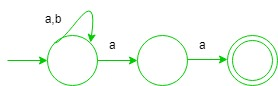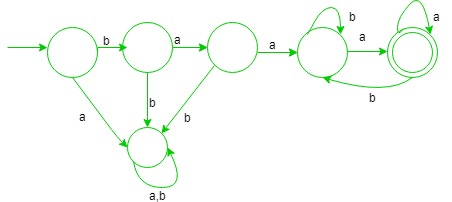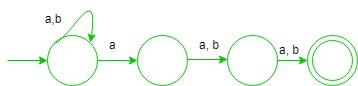Automata Theory | Set 8

• Difficulty Level : Medium
• Last Updated : 25 Feb, 2019

These questions for practice purpose for GATE CS Exam.

Ques-1: Which one of the following language is Regular?

(A) {wxwR | w,x ∈ (a+b)+}
(B) {wxwR | w ∈ (a+b)*, x ∈ {a,b}}
(C) {wwRx | w,x ∈ (a+b)+}
(D) {wwR | w ∈ (a+b)*}

Explanation:

• (A) It is correct, since this language can form regular expression which is {{ a(a + b)+a } + {b(a + b)+b}}, i.e., start and end with same symbol.
• (B) It is deterministic context free language since, string before and and after ‘x’ are same so, it is matched.
• (C) It cannot be regular since, wwR is done at first which requires comparison which cannot be done via finite automata.
• (D) It is also not regular since, comparison is required.

Option (A) is true.

Ques-2: Let w be any string of length n in {a, b}*. Consider ‘L’ be the set of all strings ending with at least n a’s. What is the minimum number of states in non deterministic finite automata that accept ‘L’?

(A) (n+3)
(B) (n+1)
(C) n
(D) 2n

Explanation:
It is correct since, the minimum number of states required for NFA for ending with at least 2 a’s is (2 + 1) i.e., regular expression will be (a + b)*aaHence, Number of states required for at least n a’s will be (n+1).

Option (B) is true.

Ques-3: What is the minimum number of states in deterministic finite automata (DFA) for string starting with ba2 and ending with ‘a’ over alphabet {a, b}?

(A) Ten
(B) Nine
(C) Eight
(D) Six

Explanation:In the above DFA, minimum number of states required is six.

Option (D) is correct.

Ques-4: Consider the following statements:

S1 = {(an)m | n = 0}
S2 = {anbn | n>=1} U {anbm | n>=1, m>=1}

Which one of the following is regular?

(A) only S1
(B) only S2
(C) both S1 and S2
(D) none

Explanation:
Both given languages are regular. Option (C) is correct.

Ques-5: What is the number of states in minimal NFA(non deterministic finite automata), which accepts set of all strings in which the third last symbol is ‘a’ over alphabet {a, b}?

(A) three
(B) four
(C) six
(D) five

Explanation:In the above NFA, minimum number of states required is four.

Option (B) is true.

My Personal Notes arrow_drop_up